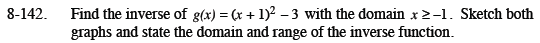Home > CCA2 > Chapter 8 > Lesson 8.3.2 > Problem8-142

8-142.

Find the inverse of g(x) = (x + 1)2 − 3 with the domain x ≥ −1. Sketch both graphs and state the domain and range of the inverse function. Homework Help ✎Let g(x) = y. Then interchange x and y.
x = (y + 1)2 − 3

Add 3 to both sides of the equation.
x + 3 = (y + 1)2

Take the square root of both sides.

$g^{-1}(x)= \sqrt{x + 3} - 1$